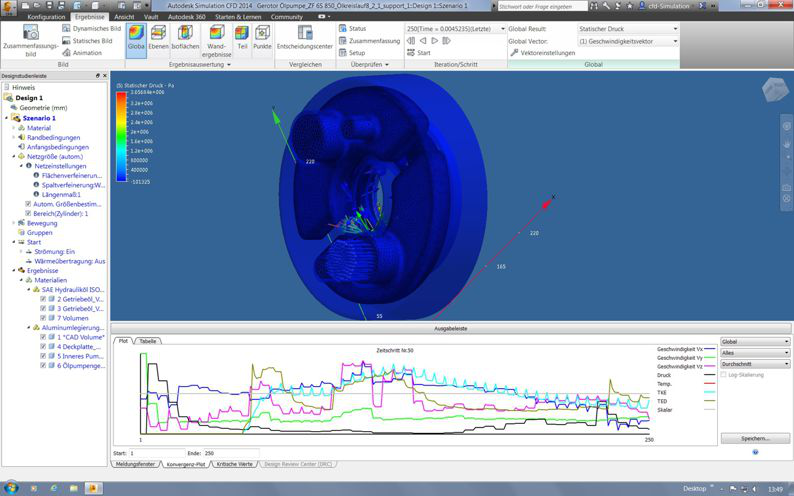FEM- Flow

 FEM-System: Ansys Workbench V12.1 Autodesk CFD-Simulation 2014The Computational Fluid Dynamics, which is also known by the acronym CFD (computational fluid dynamics) is an established method of fluid mechanics. She has the goal of fluid mechanical problems iteratively solved with numerical methods (eg. Gaussian process). The flow equations used are called Navier-Stokes equations (momentum and mass conservation). Through simplifications and assumptions it is possible to obtain simplified flow equations as the potential or Euler equation. The Computational Fluid Dynamics provides a cost effective alternative to trial rich (eg. Wind tunnel experiments, geometry optimization) and makes it possible to analyze the flow parameters measured technically not measurable.

Complex flow analysis can be carried out only with powerful computers and sophisticated CFD software. These are particularly Ansys fluid should be used. For with flow analysis from depends on the accurate and robust mesh generation.